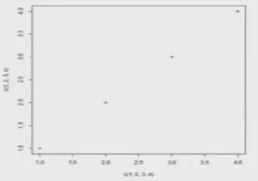# Statisticsl Functions - Correlation and Example in R Language

Descriptive Statistics :

First hand tools which gives first hand information.
• Central tendency of data
• Variation in data
• Structure and shape of data tendency
• Relationship study (correlation coefficient, rank correlation, correlation ratio, regression etc.)
Bivariate Data

Quantitative measures provide quantitative measure of relationship.

Graphical plots provide first hand visual information about the nature and degree of relationship between two variables.

Relationship can be linear or nonlinear.

x, y : Two data vectors

Data    x = (x1,x2,....,xn)                       y = (y1,y2,...,yn)

cov (x,y) :    covariance between x and y
var (x)Variance of x

Correlation coefficient

Measures the degree of linear relationship between the two variables.
cor (x,y) : correlation between x and y

Example :-

Covariance:Example :-

Correlation coefficient:
Exact positive linear dependence

> cor ( c(1,2,3,4) , c(1,2.3,4)  )
  1

Data on Daily water Demand